# Random-Approx 2013

Last week I attended the Random-Approx conference at Berkeley. I missed quite a few talks as I was also settling in my new office for the semester at the Simons Institute so I will just report on the three invited talks:

Luca Trevisan gave a talk on spectral graph theory. He first went through the basics: the eigenvalues of the normalized Laplacian(where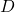is the diagonal matrix of degrees and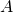the adjacency matrix of the graph) tells you something about connectivity in the graph, precisely the eigenvaluehas multiplicity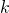if and only if the graph hasconnected components, and the largest eigenvalue is equal to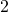if and only if the graph has a bipartite connected component (both statement are really easy to prove). This is a very nice result but a much more powerful statement would be a ‘soft’ version where one says something useful about the graph when say some eigenvalue is close to(instead of being exactly). Luckily this has been extensively studied. A famous example is the Cheeger’s inequality which states that a small second eigenvalueimplies a small expansion (the expansion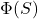of a set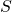of vertices is the probability that a random neighbor of a random vertex inis outside of), precisely there existssuch thatAnother example is the result of Arora, Barak and Steurer which says that a small value for theeigenvalueimplies that there is a set of roughly sizewith small expansion.

One thing that I find fascinating is that as far as I understand we still have pretty much no idea of what ‘type’ of properties are encoded in the spectrum of a graph. Of course there is a good reason for this: computing the spectrum is computationally easy so this question is directly related to asking what properties of a graph are easy to compute, and this latter question is far far beyond our current knowledge. One example of a highly non-trivial property which is ‘hidden’ in the spectrum (and the corresponding eigenbasis) is a Szemeredi decomposition. Recall that the Szemeredi regularity lemma states roughly the following: fix a precision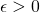, then there existssuch that for any graph on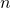vertices, withlarge enough, one can partition the vertices intoclusters of equal sizes, such that for most pairs of clusters(except a fractionof the pairs), the graph of edges between clusterand clusterlooks like a random graph (in a certain sense and up to an error of) with a certain density of edges. Another way to put it is that any large graph of sizecan be viewed as a small graph of size(withindependent of!!!!) with weighted edges. You can find the precise statement of this result here together with a proof based on the spectrum of the graph.

Santosh Vempala gave a talk on high-dimensional sampling algorithms. I already blogged about this since he gave the same talk at ICML, see here.

Persi Diaconis gave a very nice board talk about how to use algorithms to prove theorems in probability theory. Of course the standard approach is rather to go the other way around and use probability to derive new algorithms! I discussed a somewhat similar topic in the early days of this blog when I suggested that one could use results in statistics to prove new theorems in probability theory (again one usually do the opposite), see here. In his talk Diaconis illustrated the power of algorithms as a proof method for probability statements with the following problem. Letbe the set of partitions of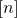. The cardinality ofis known as the Bell number. For various reasons one can be interested in computing quantities of the form

(1)for some function. Apparently there is a huge litterature that shows that such statistics can be expressed as polynomial functions of the Bell numbers. For eachone has to work hard to prove the expression. What Diaconis did in this paper is to prove an existence theorem for such an expression for ‘reasonable’ functions. Of course one can view (1) as the expectation of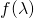when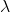is a set partition drawn uniformly at random. The key for the proof is then to have a ‘convenient algorithm’ to generate such a set partition at random, which in turns will make the analysis easy. The algorithm that they used is the following beautiful strategy of Stam. First one has to know the Dobinski’s formula:(Yup that’s right, it’s an amazing formula!) Now pick an integerat random with probability(this defines a probability distribution thanks to the Dobinski’s formula). Next droplabeled balls intoboxes. This gives a partition of, and the distribution is uniform. The point is that there are many possible algorithms to generate a partition at random, but this particular clever algorithm leads to a new and interesting point of view to prove statements about uniformly drawn set partitions.

P.S: In the last post I gave links to the videos for COLT 2013 and ICML 2013, in case you are interested here are the direct links to my talks in these events:

Bounded regret in stochastic multi-armed bandits (COLT), paper is here.

– Multiple Identifications in Multi-Armed Bandits (ICML), unfortunately for this one the sound is not correctly synchronized so it’s probably better to just look at the paper directly.

– Here is another talk I did during the summer on an older paper, it’s aboutorder Lipschitz optimization.

This entry was posted in Random graphs, Theoretical Computer Science. Bookmark the permalink.

## 2 Responses to "Random-Approx 2013"

••